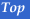## Chapter Seven: Module Summary -- Foreign Exchange and the Balance of Payments

• Foreign exchange is currency and bank deposits that are denominated in foreign money.

• The exchange rate is the amount of domestic currency that it takes to purchase one unit of foreign currency.

• From the U.S. perspective, the exchange rate can be defined as the U.S. \$ Equivalent or Currency per U.S. \$. This module uses the former definition.

• When the exchange rate rises, the dollar depreciates. Currency depreciation makes imports fall while exports rise. Conversely, when the exchange rate falls, the dollar appreciates. Currency appreciation makes imports rise while exports fall.

• The demand curve for foreign exchange is negatively sloped because as the exchange rate rises (the currency depreciates) import prices rise; therefore, the quantity of foreign exchange demanded falls.

• The supply curve for foreign exchange is positively sloped because as the exchange rate rises (the currency depreciates) export prices fall; therefore, the quanity of foreign exchange supplied rises.

• The equilibrium exchange rate is determined by the interaction of supply and demand for foreign exchange.

• The (negative) balance on goods and services or the trade deficit is the excess of imports over exports.

• A capital outflow is the purchase of a foreign asset by a domestic entity. An increase in capital outflows shifts the demand curve for foreign exchange to the right, increasing the exchange rate (depreciating the domestic currency).

• A capital inflow is the purchase of a domestic asset by a foreign entity. An increase in capital inflows shifts the supply curve for foreign exchange to the right, decreasing the exchange rate (appreciating the domestic currency).

• A country can run persistent trade deficits if it continually has capital account surpluses.

• The Balance of Payments is a record of a country's trade in goods, services, and financial assets with the rest of the world. It is composed of the current account, the capital account and the statistical discrepancy account.

• The current account primarily tracks the international flow of goods and services.

• The capital account primarily tracks the international flow of financial assets.

• In theory, current account deficits are exactly offset by capital account surpluses. However, errors and omissions in the accounts mean that the accounts do not sum to zero in practice. The statistical discrepancy account ensures that the balance of payments sums to zero.Copyright © 1996-2006 OnlineTexts.com - All Rights Reserved